# Absolute Conic

## 1. Conic

• Conic is a curve on a plance which is of form $ax^2 + bxy + cy^2 + dx + ey + f = 0$.
• Let $(x_1, x_2, x_3)^t \sim (\frac{x_1}{x_3}, \frac{x_2}{x_3}, 1)^t$ be the homogeneous coordinates of a point, then $ax^2_1 + bx_1x_2 + cx^2_2 + dx_1x_3 + ex_2x_3 + fx^2_3 = 0$.
• For matrix representation, it is of form $x^t C x = 0$.
$\begin{equation} (x_1 x_2 x_3) \left(\begin{array}{ccc} a & \frac{b}{2} & \frac{d}{2} \\ \frac{b}{2} & c & \frac{e}{2} \\ \frac{d}{2} & \frac{e}{2} & f \end{array}\right) \left(\begin{array}{c} x_1 \\ x_2 \\ x_3 \end{array}\right) = 0 \implies x^t C x = 0 \end{equation}$
• $C$ is the conic as matrix representation which is symmetric. In addition, all $kC(k \not =0)$ are the same conic.

## 2. The tangent to the conic

• Let the point $x$ be on the conic $C$ and on the line $l$. It means $x^t C x = 0$ and $l^t x = 0$. If there exists another point $y$ such as $x$, $y^t C y = 0$ and $l^t y = 0$.
• For any $\alpha > 0$, $(x + \alpha y)^t C (x + \alpha y) = x^t C x + \alpha^2 y^t C y + \alpha y^t C x + \alpha x^t C y = 0 + 0 + 0 + 0 = 0$. It implies that all the points on the line passing through points $x$ and $y$ are also on $C$, so it is a contradiction. Therefore, $l$ is the tangent to the conic and $l = Cx$.

## 3. Degenerate conic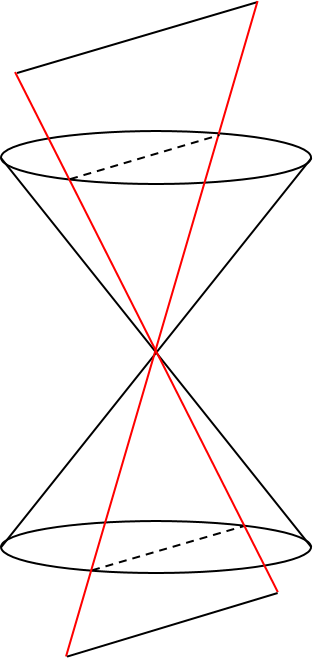• When a double conic is divided by a plane passing through its center, its cross section is two lines, not parabola, hyperbola, circle, or ellipse. These two lines $l$ and $m$ are a degenerate conic $C_{\infty}$ such that $C_{\infty} = lm^t + ml^t$.
• Since $x^t C_{\infty} x = 0$, $l^t x = 0$, and $m^t x = 0$, $C_{\infty} = lm^t + ml^t$ and $rank(C_{\infty}) = 2$.

## 4. Dual conic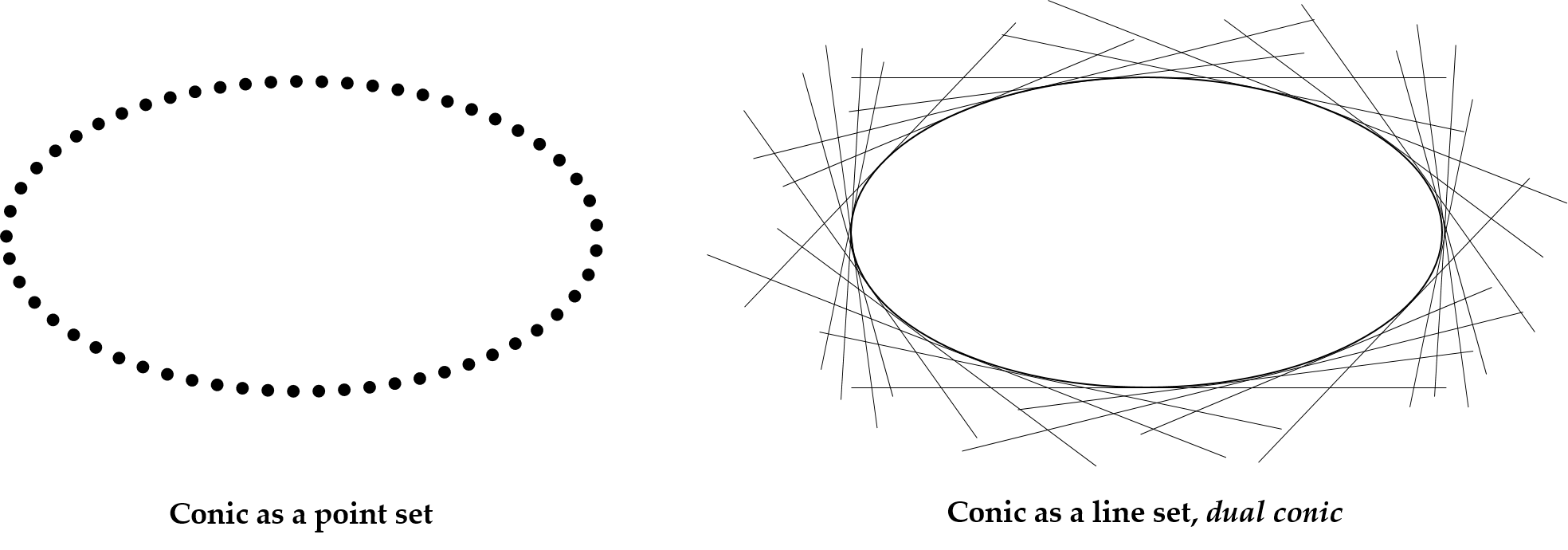• A conic on a plane is a set of points, but it can be viewed as a set of tangents to each point which is called dual conic.
• The conic $C$ satisfies $x^t C x = 0$ for a point $x$ on $C$, and the dual conic $C^{*}$ satisfies $l^t C^{*} l = 0$ for the tangent to the $x$.
• If a point $x$ is on the conic $C$, then the tangent $l$ to $x$ is $l = Cx$, so $x = C^{-1}l$.
• $x^t = l^t C^{-t} = l^t C^{-1}$ since $C$ is symmetric. It implies that $x^t C x = (l^t C^{-1})l = 0$. Therefore, $C^{*} = C^{-1}$.

## 5. Homography of conic

• Assume that a point $x$ is on the conic $C$ and a line $l$ is on the dual conic $C^{*}$ of $C$. These are transformed to $x'$, $C'$, $l'$ and $C'^{*}$ by a homography $H$.
• From $x^t C x$ and $x' = Hx$, $x'^t H^{-t} CH^{-1} x' = 0$, so $C' = H^{-t} C H^{-1}$.
• The tangent $l$ to $x$ satisfies $l^t x = 0$, so $l^t x = l^t (H^{-1}x') = (l^t H^{-1})x' = 0 = l'^t x'$. It means $l' = H^{-t}l$.
• From $l^t C^{*} l = 0$ and $l' = H^{-t}l$, $l'^t H C^{*} H^t l' = 0$, so $C'^{*} = H C^{*} H^t$.

## 6. Circular points: all the circles intersects with $l_{\infty}$ at two points

• All the circles have $b = 0$ and $a = c$ from the conic equation $ax^2_1 + bx_1x_2 + cx^2_2 + dx_1x_3 + ex_2x_3 + fx^2_3 = 0$.
• If we set $a = 1$, $x^2_1 + x^2_2 + dx_1x_3 + ex_2x_3 + fx^2_3 = 0$.
• The intersection point with $l_{\infty}$ is at infinity, so $x_3 = 0$ and it reduces to $x^2_1 + x^2_2 = 0$.
• In homogeneous coordinates, this has two solutions, $(x_1, x_2) = \{ (1, i), (1, -i) \}$.
• Therefore, the circular points are $(1, i, 0)^t$ and $(1, -i, 0)^t$.

## 7. Dual degenerate conic

• It is the dual of degenerate conic $C_{\infty} = l m^t + m l^t$.
• Degenerate conic is defined by lines, so its dual is defined by points.
• Let the circular points be $U$ and $V$, the dual degenerate conic $C^{*}_{\infty}$ satisfies $C^{*}_{\infty} = UV^t + VU^t$.
• As the dual of $x^t C x = 0$ form is $l^t C^{*} l = 0$, $C^{*}_{\infty}$ consists of the lines such that $l^t C^{*}_{\infty} l = 0$.
• $C^{*}_{\infty}$ as matrix representation is the following:
\begin{aligned} C^{*}_{\infty} &= UV^t + VU^t = \left(\begin{array}{c} 1 \\ i \\ 0 \end{array}\right) \left(\begin{array}{ccc} 1 & -i & 0 \end{array}\right) + \left(\begin{array}{c} 1 \\ -i \\ 0 \end{array}\right) \left(\begin{array}{ccc} 1 & i & 0 \end{array}\right) \\ &= \left(\begin{array}{ccc} 1 & -i & 0 \\ i & 1 & 0 \\ 0 & 0 & 0 \end{array}\right) + \left(\begin{array}{ccc} 1 & i & 0 \\ -i & 1 & 0 \\ 0 & 0 & 0 \end{array}\right) \equiv \left(\begin{array}{ccc} 1 & 0 & 0 \\ 0 & 1 & 0 \\ 0 & 0 & 0 \end{array}\right) = \left(\begin{array}{cc} I & \overrightarrow{0} \\ \overrightarrow{0} & 0 \end{array}\right) \end{aligned}
• $C^{*}_{\infty}$ is invariant to similarity homography. This homography $H$ is
\begin{aligned} H = \left(\begin{array}{cc} A & \overrightarrow{v} \\ \overrightarrow{0} & 1 \end{array}\right) \end{aligned}

where $AA^t = \lambda^2 I^2$. Since $C'^{*}_{\infty} = H C^{*}_{\infty} H^t$, \begin{aligned} C'^{*}_{\infty} &= \left(\begin{array}{cc} A & \overrightarrow{v} \\ \overrightarrow{0} & 1 \end{array}\right) \left(\begin{array}{cc} I & \overrightarrow{0} \\ \overrightarrow{0} & 0 \end{array}\right) \left(\begin{array}{cc} A^t & \overrightarrow{0} \\ \overrightarrow{v}^t & 1 \end{array}\right) = \left(\begin{array}{cc} A & \overrightarrow{v} \\ \overrightarrow{0} & 1 \end{array}\right) \left(\begin{array}{cc} A^t & \overrightarrow{0} \\ \overrightarrow{0} & 0 \end{array}\right) = \left(\begin{array}{cc} \lambda^2 I & \overrightarrow{0} \\ \overrightarrow{0} & 0 \end{array}\right) \\ &\equiv \left(\begin{array}{cc} I & \overrightarrow{0} \\ \overrightarrow{0} & 0 \end{array}\right) = C^{*}_{\infty} \end{aligned}

• Quadric is similar to conic and is defined in one more higher dimension than that of conic.
• Quadric $Q$ is a $4 \times 4$ symmetric matrix and $x^t Q x = 0$ for the point $x$ on $Q$.
• As $rank(C) = 3$ for a conic $C$ in general except for the degenerate conic whose $rank(C_{\infty}) = 2$, quadric $Q$ is, in general, $rank(Q) = 4$ except for the degenerate quadric whose $rank(Q_{\infty}) = 3$.

## 9. The intersection of quadric and plane is a conic

• For the non-colinear points $A$, $B$, and $C$ are on a plane $\Pi$, a point $x$ on $\Pi$ is represented as $x = uA + vB + wC$ for some $u$, $v$, $c \in \R$. In other words, $x = (A, B, C)(u, v, w)^t = M\overrightarrow{p}$ where $M$ is a $4 \times 3$ matrix and $\overrightarrow{p}$ is a $3 \times 1$ matrix.
• If $x$ is on the intersection area of a quadric $Q$ and $\Pi$, $x^t Q x = 0$ and $\overrightarrow{p}^t M^t Q M \overrightarrow{p} = 0$.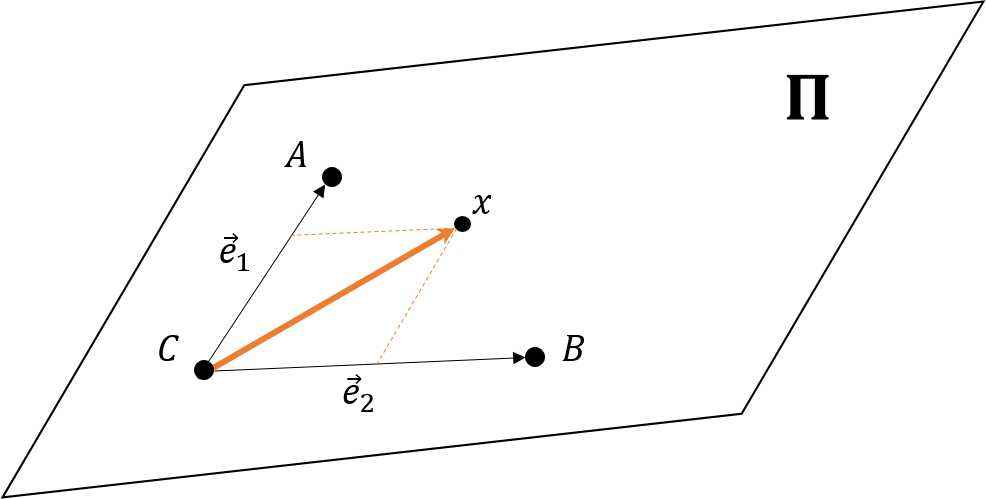• In fact, $\overrightarrow{p}$ can be represented in homogeneous coordinates which is composed of $A$, $B$, and $C$. It means that $x = m \overrightarrow{e_1} + n \overrightarrow{e_2} = m(A - C) + n(B - C) = mA + nB - (m + n)C$ where $m = u$, $n = v$, and $w = -m-n$.
• Therefore, $\overrightarrow{p}^t (M^t Q M) \overrightarrow{p}$ can be considered as the conic $C$ where $C = M^t Q M$ for the point $\overrightarrow{p}$ on $C$.

## 10. The tangent to the quadric

• For the point $x$ on the tangent plane $\Pi$ to the quadric $Q$, $x^t Q x = 0$ and $\Pi^t x = 0$. It implies that $\Pi = Q^t x = Qx$. This property is corresponding to that of conic.

• A quadric is a set of points, but it can be viewed as a set of tangent planes to each point which is called dual quadric.
• The quadric $Q$ satisfies $x^t Q x = 0$ for a point $x$ on $Q$, and the dual quadric $Q^{*}$ satisfies $\Pi^t Q^{*} \Pi = 0$ for the tangent plane to the $x$.
• The tangent plane $\Pi$ on the quadric $Q$ satisfies $\Pi = Qx$, so $x^t Q x = \Pi^t Q^{-t}Q Q^{-1}\Pi = 0$. It means $Q^{*} = Q^{-t} = Q^{-1}$. This property is corresponding to that of conic.

• Assume that a point $x$ is on the quadric $Q$ and a plane $\Pi$ is on the dual quadric $Q^{*}$ of $Q$. These are transformed to $x'$, $Q'$, $\Pi'$, and $\Pi'^{*}$ by a homography $H$.
• From $x^tQx$ and $x' = Hx$, $x'^t H^{-t} Q H^{-1} x' = 0$, so $Q' = H^{-t} Q H^{-1}$.
• From $\Pi^t Q^{*} \Pi = 0$ and $\Pi' = H^{-t} \Pi$, $\Pi'^t H Q^{*} H^t \Pi' = 0$, so $Q'^{*} = H Q^{*} H^t$.

## 13. What $Qx$ stands for where the point $x$ is NOT on the quadric $Q$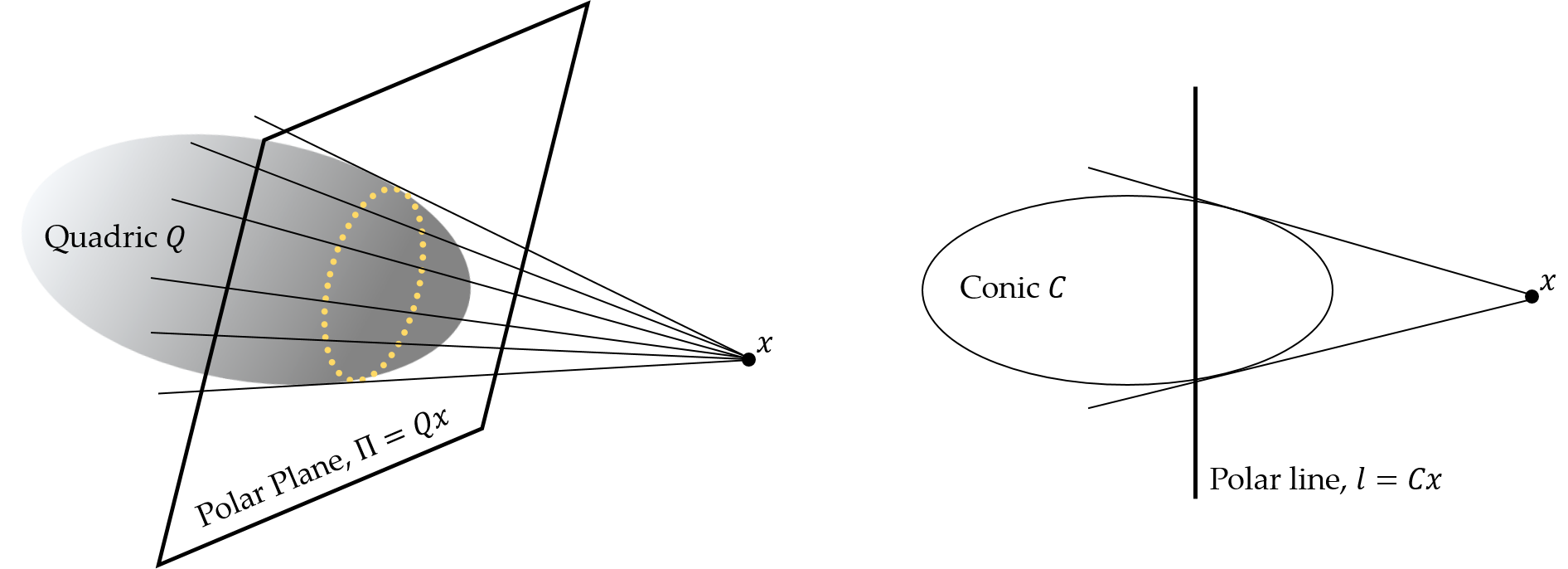• $Qx$ means a polar plane when the point $x$ is not on the quadric $Q$.
• In other words, the polar plane consists of the planes which passes through $x$ and tangent to $Q$. This property is corresponding to that of conic which is called polar line.
• Assume that the point $y$ is on $Q$ and its tangent plane $Qy$ passes through $x$. So, $(Qy)^t x = 0 = y^t Q^t x = y^t Qx$. Since $y^t Qx$ is a scalar, $y^t Qx = (y^t Qx)^t = x^t Q^t y = (Qx)^t y$. Therefore, $Qx$ is the polar plane.
• Assume that the point $y$ is on a conic $C$ and its tangent line $Cy$ passes through $x$. So, $(Cy)^t x = 0 = y^t C^t x = y^t Cx$. Since $y^t Cx$ is a scalar, $y^t Cx = (y^t Cx)^t = x^t C^t y = (Cx)^t y$. Therefore, $Cx$ is the polar line.

## 14. Absolute conic: all the spheres intersect with $\Pi_{\infty}$

• A sphere is a kind of a quadric, so the intersection of a sphere and $\Pi_{\infty} = (0, 0, 0, 1)^t$ is a conic.
• All the spheres satisfy $x^2_1 + x^2_2 + x^2_3 + d x_1 x_4 + e x_2 x_4 + f x_3 x_4 + g x^2_4 = 0$ for the point $(x_1, x_2, x_3, x_4)^t$ in homogeneous coordinates.
• The intersection with $\Pi_{\infty}$ is at infinity, so $x_4 = 0$ and it reduces to $x^2_1 + x^2_2 + x^2_3 = 0$.
• This form can be changed as $(x_1, x_2, x_3)I(x_1, x_2, x_3)^t = 0$ which is of conic form. This conic is on $\Pi_{\infty}$ and consists of the only imaginary part, which is called the absolute conic.
• The absolute conic is denoted by $\Omega_{\infty}$.
• The dual of $\Omega_{\infty}$ is called absolute dual quadric $\Omega^{*}_{\infty}$,
\begin{aligned} \left(\begin{array}{cccc} 1 & 0 & 0 & 0 \\ 0 & 1 & 0 & 0 \\ 0 & 0 & 1 & 0 \\ 0 & 0 & 0 & 0 \end{array}\right) \end{aligned}
• For all the plances $\Pi = (x_1, x_2, x_3, x_4)^t$ such that $(x_1, x_2, x_3)(x_1, x_2, x_3)^t = 0$, $\Pi$ is tangent to the $\Omega_{\infty}$. Moreover, this $\Pi$ satisfies $\Pi^t \Omega^{*}_{\infty} \Pi = 0$, which is the dual quadric form.
• Geometrically, $\Omega^{*}_{\infty}$ consists of all the tangent planes of $\Omega_{\infty}$.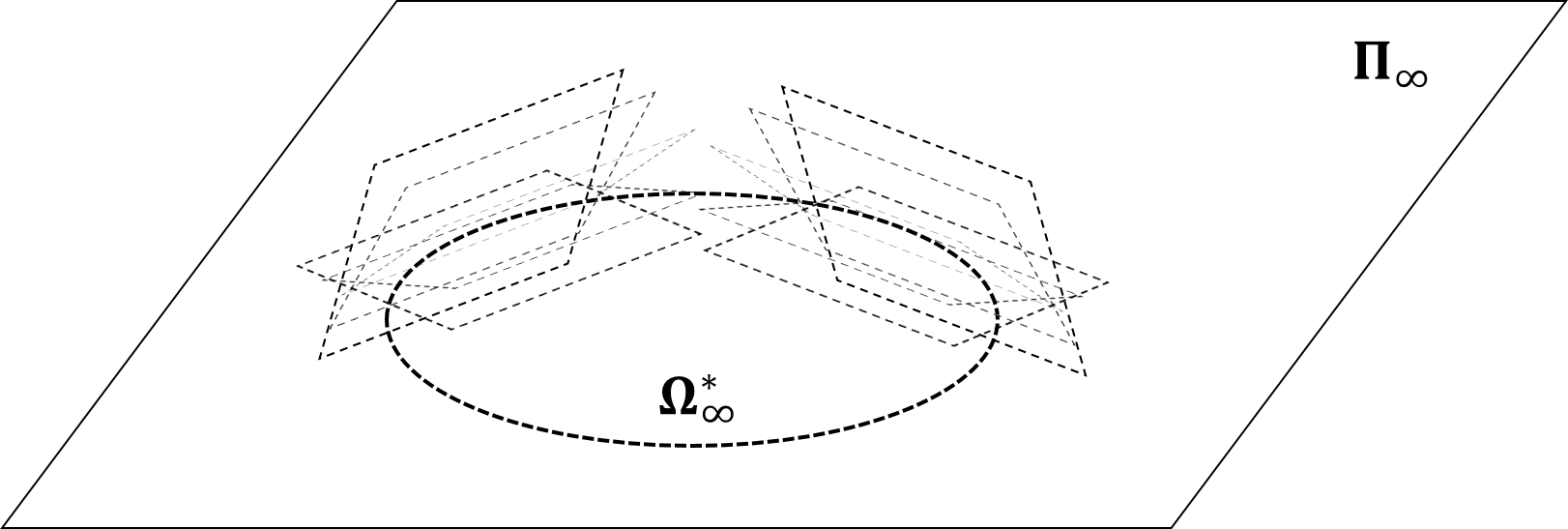## 15. Projection of $\Omega_{\infty}$

• When the point $x = (x_1, x_2, x_3, 0)^t$ on $\Pi_{\infty}$ is projected by $P$, the image of $x$ is
\begin{aligned} PX = KR[I \vert -E] \left(\begin{array}{c} x_1 \\ x_2 \\ x_3 \\ 0 \end{array}\right) = KR \left(\begin{array}{c} x_1 \\ x_2 \\ x_3 \end{array}\right) = H \overline{x} \end{aligned}

where $K$ is the intrinsic matrix of the camera, $R$ is the rotation matrix, $E$ is the camera center, $H=KR$ and $\overline{x} = (x_1, x_2, x_3)^t$. It means that $\overline{x}$ is the direction to the intersection with $\Pi_{\infty}$.

• $\Omega_{\infty}$ is defined by this $x$ which satisfies $\overline{x}^t \Omega_{\infty} \overline{x}$. Moreover, $\Omega_{\infty}$ can be transformed by this $H$ as follows:
\begin{aligned} H^{-t} \Omega_{\infty} H^{-1} &= H^{-t}IH^{-1} = (KR)^{-t} (KR)^{-1} = (R^t K^t)^{-1} (KR)^{-1} \\ &= K^{-t}R^{-t}R^{-1}K^{-1} = K^{-t}(RR^{-t})^{-1}K^{-1} = K^{-t}K^{-1} = \omega \end{aligned}
• $\overline{x}$ and $\Omega_{\infty}$ are transformed to $\omega$ and $H\overline{x}$ by $H$. Since $\overline{x}$ is on the $\Omega_{\infty}$, $H\overline{x}$ should be on $\omega$ which means $(H\overline{x})^t \omega (H\overline{x})$.
• $(H\overline{x})^t \omega (H\overline{x}) = (H\overline{x})^t (K^{-t} K^{-1}) (H\overline{x}) > 0$ yields that $K^{-t} K^{-1}$ is positive definite. Therefore, all this kind of $H\overline{x}$ are imaginary.

## 16. All the planes intersect with $\Omega_{\infty}$ at circular points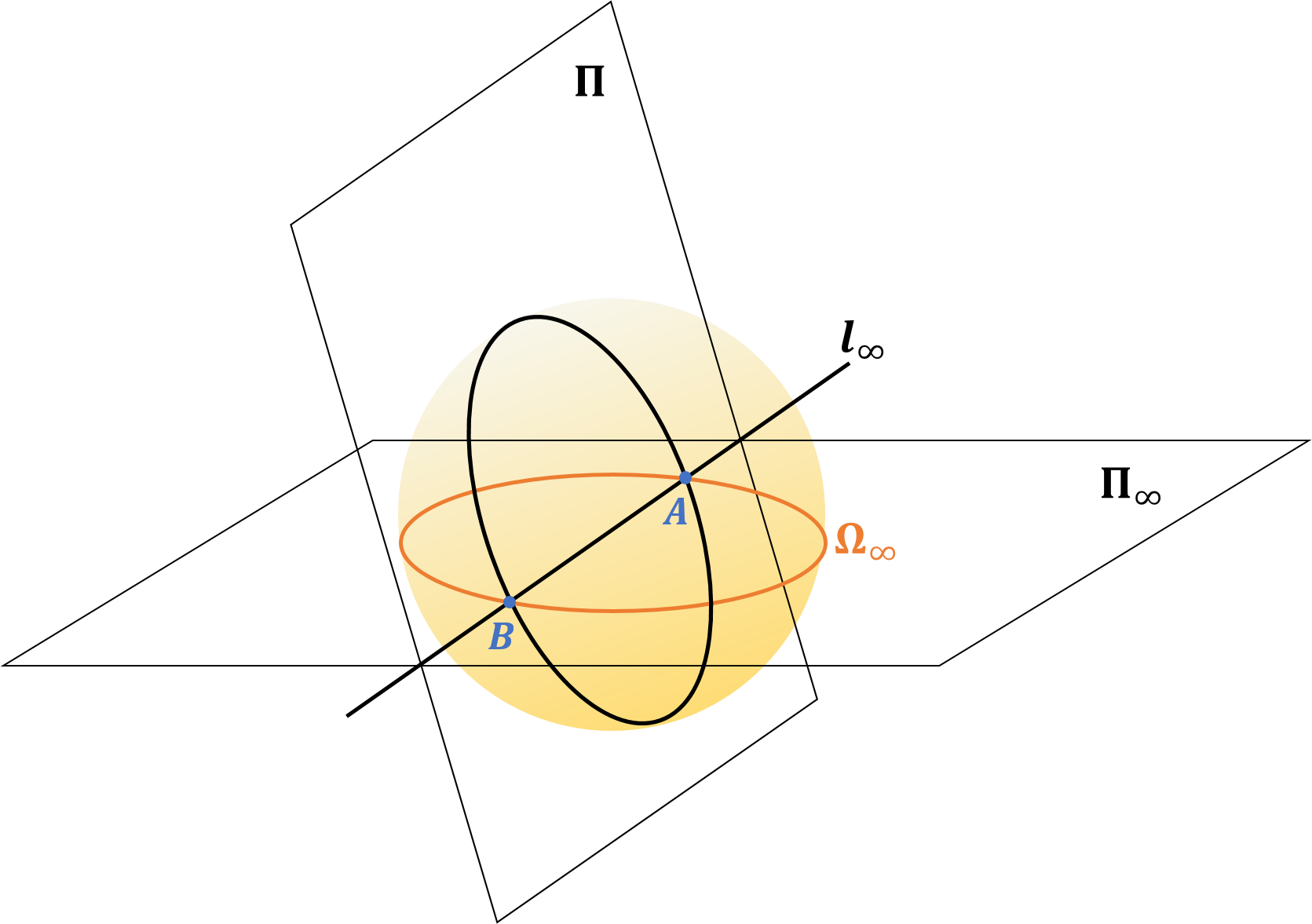• Since $\Omega_{\infty}$ is on $\Pi_{\infty}$, $l_{\infty}$ is also on $\Pi_{\infty}$. An arbitrary plane $\Pi$ includes any circles, so these circles intersect with $l_{\infty}$ at circular points. It means that this $\Pi$ includes $l_{\infty}$.
• For a circle on $\Pi$, there exists the sphere including this circle. This sphere also intersects with $\Pi_{\infty}$ because all the spheres intersect with $\Pi_{\infty}$ at absolute conic $\Omega_{\infty}$. Therefore, this sphere includes $\Omega_{\infty}$. As a result, the intersection of $\Omega_{\infty}$ and $l_{\infty}$ is circular points.

## Reference

 Hartley, R. and Zisserman, A. (2003) Multiple View Geometry in Computer Vision. 2nd Edition, Cambridge University Press, Cambridge.